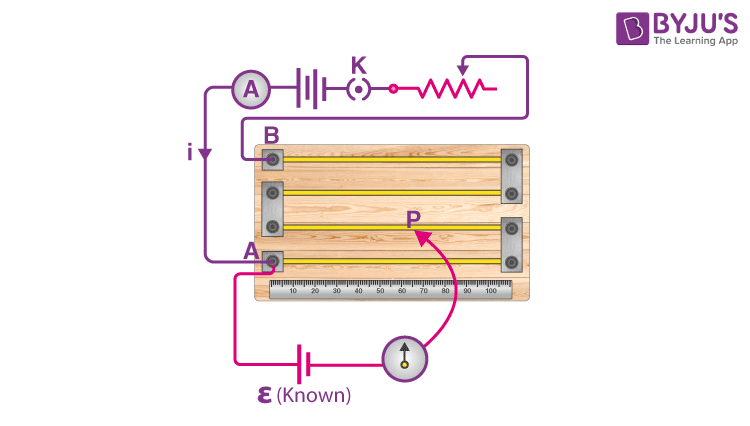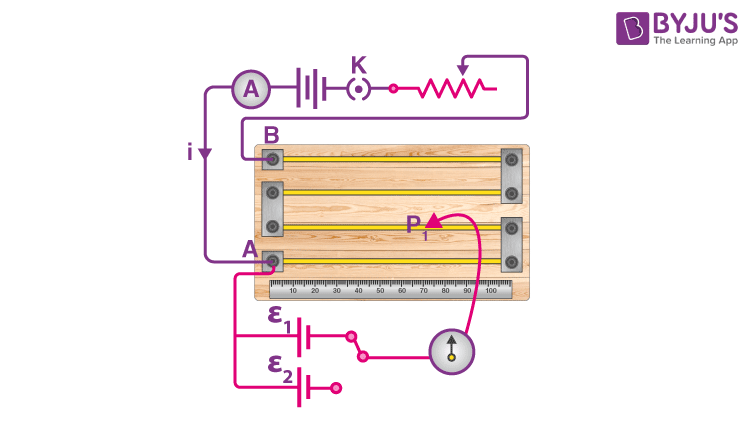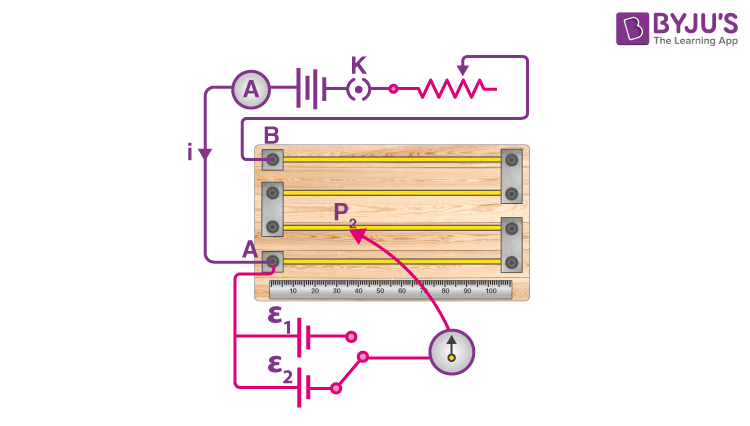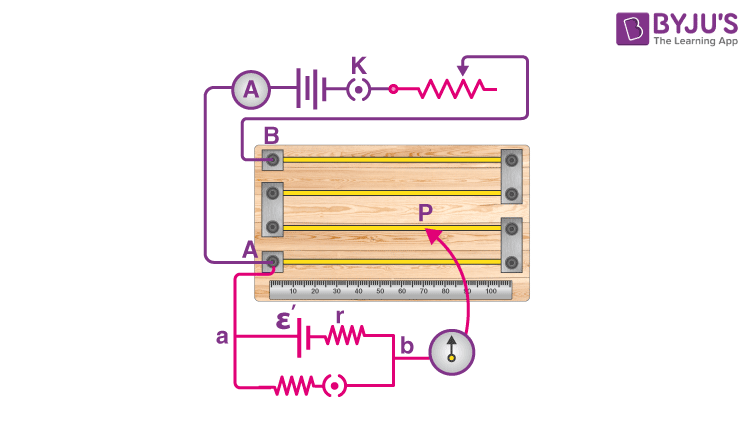Win up to 100% scholarship on Aakash BYJU'S JEE/NEET courses with ABNAT Win up to 100% scholarship on Aakash BYJU'S JEE/NEET courses with ABNAT

# Potentiometer

The potentiometer is a device that was developed in the early days of electronics development. The word ‘potentiometer’ is the combination of potential difference and metering.

## What Is a Potentiometer?

The potentiometer is basically a long piece of uniform wire across which a standard cell is connected. In the actual design, the long wire is cut into several pieces, and it is placed side by side and connected at the ends with a thick metal strip. The current flowing through the wire can be varied using a variable resistance (rheostat) connected to the circuit. The resistance can be changed manually to measure the potential difference. The potential difference between any two points in a circuit is the amount of work done in bringing the charge from the first point to the second point. When there is a potential difference, there will be a current flow in the circuit.

The potentiometer is an instrument used to measure the unknown voltage by comparing it with the known voltage. It can be used to determine the emf and internal resistance of the given cell and also used to compare the emf of different cells. The comparative method is used by the potentiometer. The reading is more accurate in a potentiometer.

## Working Principle of Potentiometer

The basic principle of the potentiometer is that the potential drop across any section of the wire will be directly proportional to the length of the wire, provided the wire is of a uniform cross-sectional area and a uniform current flows through the wire.

### To Determine the EMF of the Cell (Calibration)Let us consider that the potentiometer has a resistive wire of length ‘L’. Let one end of the wire be A and the other end B. A battery is connected to the two ends of the wire, and this forms the primary circuit. The secondary circuit is formed by connecting the end A of the wire to the cell whose emf has to be calculated, and the other terminal of the cell is connected to the galvanometer. The galvanometer is further connected to the jockey (movable point). Let ‘i’ be the current flowing through the wire.

i = ɛ/(r+R)

Here,

ɛ is the emf of the cell in the primary circuit

r is the internal resistance

R is the resistance of the wire

The voltage across the potentiometer wire of length L is taken as

VAB = V0

The fall of potential per unit length of the potentiometer wire is called the potential gradient.

Z = (V0/L) is the potential gradient.

The jockey is moved on the wire, and the null point (P) is determined. The point on the wire is called the null point when the galvanometer will not show any deflection. The length of the wire AP is taken as ‘l’.

The potential difference between A and P will be

$$\begin{array}{l}V_{A}-V_{P}=\frac{\frac{\epsilon}{(r+R)}R}{L}.l\end{array}$$

⇒VA – VP = (V0/L).l

= Z.l = Ɛ’ (since V0 = iR)

Ɛ’ is the emf of the cell connected in the secondary circuit.

### Comparison of EMF of Two cells

The potentiometer can be used to compare the emf of two cells of emf ε1 and ε2.Let P1 be the null point when cell ε1 is connected, and let ‘l1’ be the length between the end A of the wire to the null point P1. Then, the potential difference between A and P1 is

VA – VP1 = Z.l1 = ε1 ———-(1)Let P2 be the null point when the cell ε2 is connected, and let ‘l2’ be the length between the end A of the wire to the null point P2. Then, the potential difference between A and P2 is

VA – VP2 = Z.l2 = ε2 ————(2)

Comparing (1) and (2), we get

ε1 / ε2 = l1/l2

This simple mechanism thus allows one to compare the EMFs of any two sources. In practice, if one of the cells is chosen as a standard cell whose emf is known, then the emf of the other cell can be easily calculated.

### Measurement of Internal Resistance of the CellThe potentiometer can also be used to measure the internal resistance of the cell. To determine the internal resistance, the cell whose internal resistance has to be determined is connected across the resistance box through the key(K1). When the key is open, the null point is obtained at a distance l1 from A. Then,

ε = Z.l1 ——-(1)

When the key is closed, a current ‘i’ is passed through the resistance box (R). Let the null point be at a distance of l2 from A. Therefore, the potential difference will be

v = Z.l2 ——–(2)

Comparing (1) and (2), we get

ε/v = l1/l2 ———(3)

We know ε = i(r+R) and v = iR

Therefore, equation (3) becomes

[i(r+R)/ iR] = l1/l2

Therefore, internal resistance,

$$\begin{array}{l}r= R\left ( \frac{l_{1}}{l_{2}}-1\right )\end{array}$$

The potentiometer has the advantage that it draws no current from the voltage source being measured; as such, it is unaffected by the internal resistance of the source.

## Potentiometer Sensitivity

The sensitivity of the potentiometer is the slightest change in the potential difference that a potentiometer can measure. The sensitivity of the potentiometer can be increased by decreasing the potential gradient, i.e., by increasing the length of the potentiometer wire.

## Solved Examples

1) There is no current flow when the cell is connected to a 125 cm length of the potentiometer wire. If a 20 Ω resistance is connected to the cell, the balancing length is reduced to 25 cm. Determine the internal resistance of the cell.

Internal resistance, r = [(l1 – l2)/l2]R = [(125 – 100)/100] x 20 = 5 Ω

2) Let 0.2 A be the current in the primary circuit of the potentiometer. The specific resistance of the material of the wire is 40 x 10-8 Ωm, and the area of the cross-section is 0.8 x 10-6 m2. Calculate the potential gradient.

Potential gradient, Z = V/L = IR/L = Iρ/A

= [0.2 x 40 x 10-8]/0.8 x 10-6

= 0.1 volt/m

3) The length of the potentiometer wire is 40 cm. Let V1 and V2 be the potential difference for the resistance of 8Ω and 12Ω, respectively. The length of the zero deflection is obtained at point F. Find the balancing length AF.

Let Z be the potential gradient

$$\begin{array}{l}\frac{V_{1}}{V_{2}}=\frac{xl}{x(40-l)}\end{array}$$
$$\begin{array}{l}\frac{8}{12}=\frac{l}{(40-l)}\end{array}$$

l = 16 cm

4) While measuring the potential difference between the terminals of the resistance wire, the balance point is obtained at 78.4 cm. The same potential difference is obtained as 1.2 V using a voltmeter. Now, if a cell of EMF 1.018 V is used, the balancing length is 63.2 cm. What is the error in the reading of the voltmeter?

E0 = xl0

V = xl

Therefore, V = E0 l/l0

V = (1.018 x 78.4)/63.2

= 1.26 volt

Error = 1.26 – 1.2 = 0.06 Volts

5) In the primary circuit of a potentiometer, a battery of 2 V and a rheostat of 22 Ω are connected. If the length of the potentiometer is 10 m and its resistance is 18 Ω, determine the potential gradient of the wire.

Z =[ε/r + R + Rh]R/L

$$\begin{array}{l}Z = \left ( \frac{\epsilon }{r+R+R_{h}} \right )\frac{R}{L}\end{array}$$
$$\begin{array}{l}Z = \left ( \frac{2}{0+18+22} \right )\frac{18}{10}=0.09 V/m\end{array}$$

6) A cell of internal resistance 1.5 Ω and of emf 1.5 volt balances at 500 cm on the potentiometer wire. If a wire of 15 Ω is connected between the balance point and the cell, then the balance point will shift to

a) Zero

b) By 500 cm

c) By 750 cm

d) None of the above

Answer: d) None of the above

Solution:

The balancing point will not be affected by the addition of resistance between the balance point and the cell.

7) For measuring the potential difference, the potentiometer is preferred in comparison to a voltmeter because

a) Potentiometer is more sensitive than a voltmeter

b) The resistance of the potentiometer is less than the voltmeter

c) Potentiometer is cheaper than a voltmeter

d) Potentiometer will not take current from the circuit

Answer: d) Potentiometer will not take current from the circuit

8) Sensitivity of the potentiometer can be increased by

a) Increasing the emf of the cell

b) Increasing the length of the potentiometer wire

c) Decreasing the length of the potentiometer wire

d) None of the above

Answer: b) Increasing the length of the potentiometer wire

Solution:

The sensitivity of the potentiometer can be increased by decreasing the potential gradient, i.e., by increasing the length of the potentiometer wire.

Related Video## Frequently Asked Questions on Potentiometer

Q1

### What is the principle of a potentiometer?

According to the principle of a potentiometer, the potential dropped across a segment of a wire of uniform cross-section carrying a constant current is directly proportional to its length.

Q2

### What is the use of a potentiometer?

The electrical potential or EMF of the cell is measured using a potentiometer.

Q3

### Why is a potentiometer preferable over a voltmeter?

When measuring the emf of a cell, the potentiometer is preferred over the voltmeter since it is a null device that does not draw any current. Whereas, the voltmeter draws current from the cell.